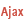ONLINE HELP WINDEV, WEBDEV AND WINDEV MOBILEVersion: 15161718192021222324252627
 Home |Sign in | EnglishHelpWLanguageWLanguage functionsControls, pages and windowsDrawing functionsPrefix syntaxDrawing functions on WDPic variablesDrawPolylinePresentationExampleCoordinatesRelated examplesSee alsoOpenSaveAlphaBlendBackgroundChangeModeDrawArcDrawChordDrawCircleDrawLineDrawPointDrawPolygonDrawPolylineDrawRectangleDrawRectangleGradientDrawRoundedRectangleDrawSliceDrawTextDrawTextRTFEndDrawingFillFontHorizontalSymmetryInvertColorMergeLayerPixelColorPixelOpacityResizeRotationSaveStartDrawingVerticalSymmetryPicMergeLayerPicOpenPicResizePicRotationPicSave
• Coordinates
WINDEVWEBDEVWINDEV MobileOthers<WDPic variable>.DrawPolyline (Function)
In french: <Variable WDPic>.DessinePolyligneNot available
Draws a line composed of multiple segments in a variable of type WDPic.
The first coordinate indicates the starting point of the first segment, then each coordinate indicates the end of the previous segment and the beginning of the next segment.
Example
MyWDPicImage is WDPic = "Test.gif"

MyWDPicImage.Pen(LightBlue, 2, 4)
arrCoordinatesXY2 is array of 0 int = [2, 2, 140, 2, 150, 70, 0, 100, 200,100]
MyWDPicImage.DrawPolyline(arrCoordinatesXY2)

IMG_MyDrawing = MyWDPicImage
Syntax

Building a line point by point

<WDPic image>.DrawPolyline(<NbPoint> , <X1> , <Y1> , <X2> , <Y2> [, <Xn> [, <Yn> [, <Line color>]]])
<WDPic image>: WDPic variable
Name of the WDPic variable to be used. Only the background layer will be handled.
<NbPoint>: Integer
Number of line segments. This parameter defines the number of <X>, <Y> pairs to specify in the function.
<X1>: Integer
X-coordinate of the first segment of the line. These coordinates are expressed in pixels.
<Y1>: Integer
Y-coordinate of the first segment of the line. These coordinates are expressed in pixels.
<X2>: Integer
X-coordinate of the second segment of the line. These coordinates are expressed in pixels.
<Y2>: Integer
Y-coordinate of the second segment of the line. These coordinates are expressed in pixels.
<Xn>: Optional integer
X-coordinate of the N segment of the line. These coordinates are expressed in pixels.
<Yn>: Optional integer
Y-coordinate of the N segment of the line. These coordinates are expressed in pixels.
<Line color>: Integer or constant (optional)
Line color. This color can correspond to:
If this parameter is not specified, the line color:

Building a line via an array of X and Y-coordinates

<WDPic image>.DrawPolyline(<Array of coordinates> [, <Line color>])
<WDPic image>: WDPic variable
Name of the WDPic variable to be used. Only the background layer will be handled.
<Array of coordinates>: Array of integers or array of points
WLanguage array of integers containing the coordinates of segments that make up the line. The even indices represent the X-coordinates while the odd indices represent the Y-coordinates of the points. These coordinates are expressed in pixels.
<Line color>: Integer or constant (optional)
Line color. This color can correspond to:
If this parameter is not specified, the line color:Versions 26 and laterBuilding a line using a variable of type Polygon

<WDPic image>.DrawPolyline(<Polygon> [, <Line color>])
<WDPic image>: WDPic variable
Name of the WDPic variable to be used. Only the background layer will be handled.
<Polygon>: Polygon variable
Name of the Polygon variable that describes the different points of the polygon.
<Line color>: Integer or constant (optional)
Line color. This color can correspond to:
If this parameter is not specified, the line color:New in version 26Building a line using a variable of type Polygon

<WDPic image>.DrawPolyline(<Polygon> [, <Line color>])
<WDPic image>: WDPic variable
Name of the WDPic variable to be used. Only the background layer will be handled.
<Polygon>: Polygon variable
Name of the Polygon variable that describes the different points of the polygon.
<Line color>: Integer or constant (optional)
Line color. This color can correspond to:
If this parameter is not specified, the line color:Building a line using a variable of type Polygon

<WDPic image>.DrawPolyline(<Polygon> [, <Line color>])
<WDPic image>: WDPic variable
Name of the WDPic variable to be used. Only the background layer will be handled.
<Polygon>: Polygon variable
Name of the Polygon variable that describes the different points of the polygon.
<Line color>: Integer or constant (optional)
Line color. This color can correspond to:
If this parameter is not specified, the line color:
Remarks

Coordinates

Coordinates are specified with respect to the upper-left corner of the image (coordinates: (0.0)).
Related Examples:Unit examples (WINDEV): The drawing functions [ + ] Using the main drawing functions of WINDEV to:- Initialize an Image control for drawing- Draw simple shapes- Write a text into a drawing- Change the color in a drawing
Business / UI classification: Neutral code
Component: wd270pnt.dll
Minimum version required
• Version 25